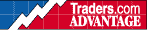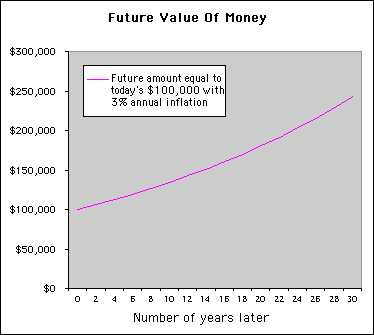HOT TOPICS LIST

INDICATORS LIST

LIST OF TOPICS

# Time Value Of Money

07/10/00 02:51:27 PM
by Han Kim

How much money do you really have? Over time inflation will decrease the value of your investment and your profit may not be as large as anticipated.

Security:   N/A
Position:   N/A

 One factor that is not often taken into consideration when calculating rates of return is inflation. This means that a dollar today is worth less than a dollar in the future. Let's assume that the inflation is 3% annually (3% is the average inflation rate for the last 10 years). Supose you invested \$100,000 ten years ago and the value of your investment grew to \$125,000. Thinking that you made a profit of \$25,000, you redeem your investment. In reality, at a 3% inflation rate, your \$100,000 needs to be \$134,392 to have the same purchasing power as you did with \$100,000 ten years ago. Therefore your 25% gain is actually a loss of \$9,392. The breakeven point is calculated using the following formula:Future Worth = Present Worth * (1 + Inflation rate) ^ Number of yearsF = P(1 + i)NWe'll use the \$100,000 investment we've been discussing as an example:Future Worth = \$100,000 * (1 + 0.03) ^ 10Future Worth = \$134,392If your investment will return greater than this breakeven point, you will have beat inflation and actually made a profit. However, if your investments will not return as much, then you should consider an alternative investment.The chart below displays the equivalent future dollars to equal today's \$100,000 over a 30-year period.. Graphic provided by: Microsoft Excel. As you watch your investments grow, you can factor in inflation and get a more realistic idea of your profits.

Han Kim

 Comments or Questions? Article Usefulness 5 (most useful) 4 3 2 1 (least useful)